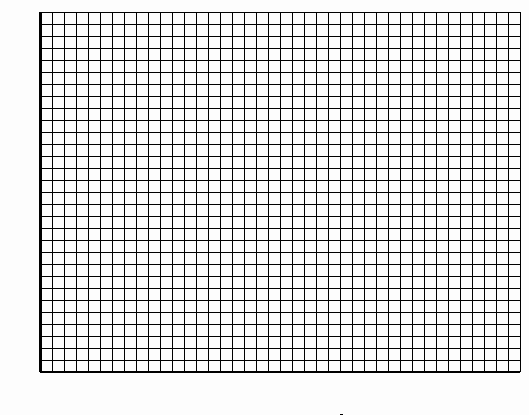PRINT THIS ANSWER SHEET TO FILL OUT AND TURN IN

NAME_____________________________ CLASS________________DATE___________

FoilSim

Lift Relationships Worksheet

Using FoilSim, set the following parameters:

Airspeed: 100 mph
Altitude: 0 ft
Angle: 0.0 degrees
Thickness: 12.5%
Camber: 0.0
Area: 1 sq ft.

1. What is the value of lift at the original parameters? ___________

2. Determine the lift value for each of the prescribed Angle levels, while keeping the other values the same.

 Angle Lift (lb.) 0 degrees _______________ 2 degrees _______________ 4 degrees _______________ 8 degrees _______________ 16 degrees ________________

3. On the graph below, graph the values for Angle and Lift. (Place the Angle on the y-axis and the Lift on the x-axis.)4. What is the relationship between angle and lift? ___________________________

5. Write an equation that will sum up this relationship. ________________________

6. Using FoilSim, and keping the angle set at 4 degrees, determine the Lift values for the following Airspeeds.

 Airspeed Lift (lb.) 25 mph ________________ 50 mph ________________ 100 mph ________________ 200 mph _________________

7. On the graph below, graph the values for Airspeed and the corresponding Lift levels.8. What is the relationship between the airspeed and the lift? _____________________________

9. Write an equation that will sum up this relationship. __________________________________

Extra Activity:

a. Using FoilSim, manipulate the values for all the different parameters until you get the highest lift value possible on this particular simulation.

b. What were your values for the different parameters?

 Airspeed Altitude Angle Thickness Camber Area Lift __________ ___________ ___________ ___________ ___________ ___________ ___________

c. What do you think will be the result if this lift was applied to a real plane?